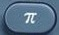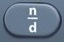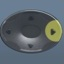# Cylinder

Cylinder = Three-Dimensional Shape with Circular Top and BaseBasics
r = radius = distance halfway across circular top or base
d = diameter = distance all the way across circular top or base
h = height
SA = Surface Area = area covering surface, including top and base, of cylinder
LA = Lateral Area = area covering surface, excluding top and base, of cylinder
V = Volume = space occupied by cylinder

Formulas (Mathematics Formula Sheet)

SA = 2πrh+ 2πr²

V = πr²h

Formulas (NOT on Mathematics Formula Sheet)

LA = 2πrh

r =$\bf\displaystyle \frac{1}{2}d$

Question
A can of soda has a radius of 3 inches and height 6 inches.  What is its surface area?

54π square inches

SA = 2πrh+ 2πr²
SA = 2 × π × 3 × 6+ 2 × π × 3² = 54π

Input Display Commentblinker clears screen
2 ×× 3 × 6
+

2 ×× 32*π*3*6+2*π*3² SA = 2πrh + 2πr²54π Answer
###### Cylinder

Question
With reference to the question above, what is the area of the can in decimal form?

169.6460033 square inches

See Toggle.
54π ⇔ 169.6460033

Input Display Commentblinker clears screen
2 ×× 3 × 6
+

2 ×× 32*π*3*6+2*π*3² SA = 2πrh + 2πr²54π169.6460033 Answer
(Toggle)
###### Cylinder

Question
With reference to the question above, what is the area of the can to the nearest tenth?

169.6 square inches

See Rounding.
169.6460033 → 169.6

Question

A can of soda has a radius of 3 inches and height 6 inches.  To the nearest tenth of a square foot, what is its surface area?

1.2 square feet

See Measurement.
Square Feet = Square Inches ÷ 144 square inches per square foot
Square Feet = 169.6460033  ÷ 144 = 1.178097245 = 1.2 after rounding

Input Display Commentblinker clears screen
2 ×× 3 × 6
+

2 ×× 32*π*3*6+2*π*3² SA = 2πrh + 2πr²54π169.6460033
169.6460033 ÷ 144 169.6460033÷144 Square Feet = Square Inches ÷ 144 square inches per square foot1.178097245 Answer
###### Cylinder

Question
A can of soda has a radius of 4 inches and height 8 inches.  To the nearest hundredth of a cubic inch, what is its volume?

402.12 cubic inches

V = πr²h
V = π × 4² × 8 = 128π = 402.1238597 after toggle = 402.12 afterounding

Input Display Commentblinker clears screen× 4× 8 π*4²*8 V = πr²h128π402.1238597 Answer
(before rounding)
###### Cylinder

Question
A can of soda has a diameter of$\bf\displaystyle8\frac{1}{2}$ inches and height$\bf\displaystyle9\frac{1}{4}$ inches.  To the nearest hundredth of a cubic inch, what is its volume?

524.89 cubic inches

r =$\bf\displaystyle \frac{1}{2}d$

r =$\bf\displaystyle\frac{1}{2}\times8\frac{1}{2}$ =$\bf\displaystyle\frac{17}{4}$

V = πr²h

V = π x$\bf\displaystyle\frac{17}{4}^{2}\times9\frac{1}{4}$ =$\bf\displaystyle\frac{10693\pi }{64}$ = 524.8914101 after toggle = 524.89 after rounding

Input Display Commentblinker clears screen12×812$\bf\displaystyle\frac{1}{2}*8\frac{1}{2}$ r =$\bf\displaystyle \frac{1}{2}d$$\bf\displaystyle\frac{17}{4}$  r×174×914π*$\bf\displaystyle\frac{17}{4}^{2}*9\frac{1}{4}$ V = πr²h$\bf\displaystyle\frac{10693\pi }{64}$524.8914101 Answer
(before rounding)
###### Cylinder

Question
A can of soda has a radius of 3 inches and height 6 inches.  To the nearest tenth of a square inch, what is its lateral area?

113.1 square inches

LA = 2πrh
LA = 2 x π x 3 x 6 = 36π = 113.0973355 after toggle = 113.1 after rounding

Input Display Commentblinker clears screen
2 ×× 3 × 6 2*π*3*6 LA = 2πrh36π113.0973355 Answer
(before rounding)
###### Cylinder

Practice – Questions
1.  A canister has a radius of 5 inches and height 7 inches.  To the nearest tenth of square inch, what is its surface area?

2.  With reference to Question 2, what is the canister’s surface area to the nearest tenth of a square foot?

3.  A canister has a radius of 5 inches and height 7 inches.  To the nearest hundredth of a cubic inch, what is its volume?

4.  A canister has a diameter of$\bf\displaystyle10\frac{1}{2}$ inches and height$\bf\displaystyle7\frac{1}{4}$ inches.  To the nearest hundredth of a cubic inch, what is its volume?

5.  A canister has a radius of 5 inches and height 7 inches.  To the nearest tenth of a square inch, what is its lateral area?